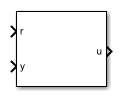# RST Controller

Predictive control using a polynomial representation

•Libraries:
Simscape / Electrical / Control / General Control

## Description

The RST Controller block implements a generalized predictive controller using a reference signal tracking polynomial representation. The diagram shows the equivalent circuit for the control algorithm.### Equations

A controlled auto-regressive integrated moving average (CARIMA) model describes the plant:

`$A\left({z}^{-1}\right)y\left(k\right)={z}^{-d}B\left({z}^{-1}\right)u\left(k-1\right)+\frac{e\left(k\right)C\left({z}^{-1}\right)}{D\left({z}^{-1}\right)}$`

`$A\left({z}^{-1}\right)=1+{a}_{1}{z}^{-1}+\cdots +{a}_{{n}_{A}}{z}^{-{n}_{A}}$`

`$B\left({z}^{-1}\right)={b}_{0}+{b}_{1}{z}^{-1}+\cdots +{b}_{{n}_{B}}{z}^{-{n}_{B}}$`

`$C\left({z}^{-1}\right)=1$`

`$D\left({z}^{-1}\right)=1-{z}^{-1},$`

where:

• d is the system dead-time.

• y(k) is the plant output.

• u(k) is the controller output.

• e(k) is white noise with a zero-mean value.

• A(z-1) and B(z-1) are the system polynomials.

• nA and nB are the polynomials degrees.

• C(z-1) and D(z-1) are the disturbance polynomials for obtaining the steady-state error.

The prediction model is given as

`$\stackrel{^}{y}\left(k+j|k\right)={G}_{j-d}\left({z}^{-1}\right)D\left({z}^{-1}\right){z}^{-d-1}u\left(k+j\right)+\frac{{H}_{j-d}\left({z}^{-1}\right)D\left({z}^{-1}\right)}{C\left({z}^{-1}\right)}u\left(k-1\right)+\frac{{F}_{j-d}\left({z}^{-1}\right)}{C\left({z}^{-1}\right)}y\left(k\right)$`

and

`$j=\overline{hi,hp}$`

where:

• hi is the minimum prediction.

• hp is the prediction horizon.

The future control sequence, computed at time k, is

where

and hc is the control horizon.

The predicted values of the output is

To determine the system polynomials, ${F}_{j-d}\left({z}^{-1}\right)$, ${G}_{j-d}\left({z}^{-1}\right)$, and ${H}_{j-d}\left({z}^{-1}\right)$, the block uses two Diophantine equations. The first Diophantine equation is

`$\frac{C\left({z}^{-1}\right)}{A\left({z}^{-1}\right)D\left({z}^{-1}\right)}={E}_{j-d}\left({z}^{-1}\right)+{z}^{-j+d}\frac{{F}_{j-d}\left({z}^{-1}\right)}{A\left({z}^{-1}\right)D\left({z}^{-1}\right)},$`

where:

`${E}_{j-d}\left({z}^{-1}\right)=1+1+{e}_{1}{z}^{-1}+\cdots +{e}_{{n}_{E}}{z}^{-{n}_{E}}$`

`${F}_{j-d}\left({z}^{-1}\right)={f}_{0}+{f}_{1}{z}^{-1}+\cdots +{f}_{{n}_{F}}{z}^{-{n}_{F}}$`

`${n}_{E}=j-d-1$`

`${n}_{F}=\text{max}\left({n}_{A}+{n}_{D}-1,{n}_{C}-j+d\right)$`

The second Diophantine equation is

`${E}_{j-d}\left({z}^{-1}\right)B\left({z}^{-1}\right)=C\left({z}^{-1}\right){G}_{j-d}\left({z}^{-1}\right)+{z}^{-j+d}{H}_{j-d}\left({z}^{-1}\right),$`

where:

`${G}_{j-d}\left({z}^{-1}\right)={g}_{0}+{g}_{1}{z}^{-1}+\cdots +{g}_{{n}_{G}}{z}^{-{n}_{G}}$`

`${H}_{j-d}\left({z}^{-1}\right)={h}_{0}+{h}_{1}{z}^{-1}+\cdots +{h}_{{n}_{H}}{z}^{-{n}_{H}}$`

`${n}_{G}=j-d-1$`

`${n}_{H}=\text{max}\left({n}_{C},{n}_{B}+d\right)-1$`

The resulting prediction model is

`$\stackrel{^}{y}\left(k+j|k\right)={G}_{j-d}\left({z}^{-1}\right)D\left({z}^{-1}\right){z}^{-d-1}u\left(k+j\right)+{\stackrel{^}{y}}_{0}\left(k+j|k\right),$`

where

`${\stackrel{^}{y}}_{0}\left(k+j|k\right)=\frac{{H}_{j-d}\left({z}^{-1}\right)D\left({z}^{-1}\right)}{C\left({z}^{-1}\right)}u\left(k-1\right)+\frac{{F}_{j-d}\left({z}^{-1}\right)}{C\left({z}^{-1}\right)}y\left(k\right)$`

represents the free response of the system.

Using the matrix notation, the prediction model can be written as

`$\text{y^}={\text{Gu}}_{d}+{\text{y^}}_{0},$`

where:

`$\text{y^}={\left[\stackrel{^}{y}\left(k+hi|k\right),\stackrel{^}{y}\left(k+hi+1|k\right),\cdots ,\stackrel{^}{y}\left(k+hp|k\right)\right]}^{T}$`

`$\text{G}=\left[\begin{array}{cccccc}{g}_{hi-d-1}& \cdots & {g}_{0}& 0& \cdots & 0\\ {g}_{hi-d}& \cdots & {g}_{1}& {g}_{0}& \cdots & 0\\ \cdots & \cdots & \cdots & \cdots & \cdots & \cdots \\ {g}_{hc-1}& \cdots & \cdots & \cdots & \cdots & {g}_{0}\\ {g}_{hp-d-1}& \cdots & \cdots & \cdots & \cdots & {g}_{hp-hc-1}\end{array}\right]$`

`${\text{u}}_{d}={\left[D\left({z}^{-1}\right)u\left(k\right),\cdots ,D\left({z}^{-1}\right)u\left(k+hc-1\right)\right]}^{T}$`

`${\text{y^}}_{0}={\left[{\stackrel{^}{y}}_{0}\left(k+hi|k\right),{\stackrel{^}{y}}_{0}\left(k+hi+1|k\right),\cdots ,{\stackrel{^}{y}}_{0}\left(k+hp|k\right)\right]}^{T}$`

To minimize tracking error and controller output, the block uses a cost function. To trade off between the minimization of the tracking error and the minimization of the controller output, the block uses a weighting factor, λ, such that

`$J={\left({\text{Gu}}_{d}+{\text{y^}}_{0}-\text{w}\right)}^{T}\left({\text{Gu}}_{d}+{\text{y^}}_{0}-\text{w}\right)+\lambda {\text{u}}_{d}^{T}{\text{u}}_{d}$`

for

`$D\left({z}^{-1}\right)u\left(k+i\right)=0$`

and

`$i\in \left[hc,hp-d-1\right],$`

where w is the reference trajectory vector. Minimizing the cost function, yields the equation for the optimal control sequence:

`${\text{u}}_{d}^{*}=\left({\text{G}}^{T}\text{G}+\lambda {\text{I}}_{hc}\right){\text{G}}^{T}\left[\text{w}-{\text{y^}}_{0}\right].$`

As γj and $j=\overline{hi,hp}$ are elements in the first row of the matrix ${\left({\text{G}}^{T}\text{G}+\lambda {\text{I}}_{hc}\right)}^{-1}{\text{G}}^{T}$, applying the receding horizon principle yields the control algorithm equation as

`$D\left({z}^{-1}\right)u\left(k\right)=\sum _{j=hi}^{hp}{\gamma }_{j}\left[w\left(k+j\text{|}k\right)-{\stackrel{^}{y}}_{0}\left(k+j|k\right)\right].$`

Substitution using ${\stackrel{^}{y}}_{0}\left(k+j|k\right)=\frac{{H}_{j-d}\left({z}^{-1}\right)D\left({z}^{-1}\right)}{C\left({z}^{-1}\right)}u\left(k-1\right)+\frac{{F}_{j-d}\left({z}^{-1}\right)}{C\left({z}^{-1}\right)}y\left(k\right)$ yields this form of the control algorithm equation:

`$C\left({z}^{-1}\right)D\left({z}^{-1}\right)u\left(k\right)=-\sum _{j=hi}^{hp}{\gamma }_{j}{H}_{j-d}\left({z}^{-1}\right)D\left({z}^{-1}\right)u\left(k-1\right)-\sum _{j=hi}^{hp}{\gamma }_{j}{F}_{j-d}\left({z}^{-1}\right)y\left(k\right)+\sum _{j=hi}^{hp}{\gamma }_{j}C\left({z}^{-1}\right)w\left(k+j\right).$`

The polynomial form of the control algorithm follows as

`$R\left({z}^{-1}\right)u\left(k\right)+S\left({z}^{-1}\right)y\left(k\right)=T\left({z}^{-1}\right)w\left(k+hp\right),$`

where:

`$S\left({z}^{-1}\right)=\sum _{j=hi}^{hp}{\gamma }_{j}{F}_{j-d}\left({z}^{-1}\right),$`

and

`$T\left({z}^{-1}\right)=C\left({z}^{-1}\right)\sum _{j=hi}^{hp}{\gamma }_{j}{z}^{-hp+j}.$`

### Limitations

To obtain the R, S, and T polynomials, use the discrete-time instead of the continuous-time transfer function.

## Ports

### Input

expand all

Plant system reference signal.

Data Types: `single` | `double`

Plant system output signal.

Data Types: `single` | `double`

### Output

expand all

Control system output signal.

Data Types: `single` | `double`

## Parameters

expand all

Method for parameterizing the controller. If you know the discrete-time R, S, and T polynomial values, select `Controller polynomials`. Otherwise, select `Generate polynomials`.

#### Dependencies

Selecting a parameterization method enables other parameters.

Vector of the R polynomials for the RST control.

#### Dependencies

Selecting `Controller polynomials` for the Controller parameterization parameter enables this parameter.

Vector of the S polynomials for the RST control.

#### Dependencies

Selecting `Controller polynomials` for the Controller parameterization parameter enables this parameter.

Vector of the T polynomials for the RST control.

#### Dependencies

Selecting `Controller polynomials` for the Controller parameterization parameter enables this parameter.

Numerator of the system discretized transfer function. To determine the discrete transfer function, if you have a license for Control System Toolbox™, use the `c2d` function.

#### Dependencies

Selecting `Generate polynomials` for the Controller parameterization parameter enables this parameter.

Denominator of the system discretized transfer function. To determine the discrete transfer function, if you have a license for Control System Toolbox, use the `c2d` function.

#### Dependencies

Selecting `Generate polynomials` for the Controller parameterization parameter enables this parameter.

Number of samples in the control horizon.

#### Dependencies

Selecting `Generate polynomials` for the Controller parameterization parameter enables this parameter.

Weighting factor for the RST controller.

#### Dependencies

Selecting `Generate polynomials` for the Controller parameterization parameter enables this parameter.

Number of samples of the dead time.

#### Dependencies

Selecting `Generate polynomials` for the Controller parameterization parameter enables this parameter.

Time interval between samples. If the block is inside a triggered subsystem, inherit the sample time by setting this parameter to `-1`. If this block is in a continuous variable-step model, specify the sample time explicitly. For more information, see What Is Sample Time? and Specify Sample Time.

 Camacho, E. F. and C. Bordons. Model Predictive Control. Second Edition, London: Springer, 2007.